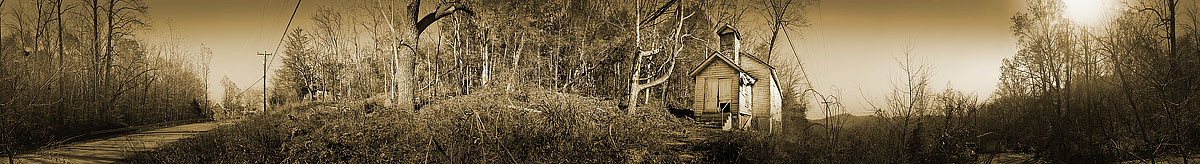O l d   M a r l   R i d g e   C h u r c h   R o c k b r i d g e   C o u n t y
N e w  T o p o g r a p h i c s  O f  O l d  V i r g i n i a